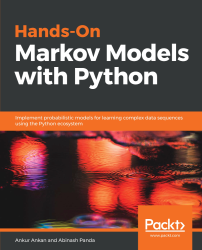•#### Hands-On Markov Models with Python#### Overview of this book

Hidden Markov Model (HMM) is a statistical model based on the Markov chain concept. Hands-On Markov Models with Python helps you get to grips with HMMs and different inference algorithms by working on real-world problems. The hands-on examples explored in the book help you simplify the process flow in machine learning by using Markov model concepts, thereby making it accessible to everyone. Once you’ve covered the basic concepts of Markov chains, you’ll get insights into Markov processes, models, and types with the help of practical examples. After grasping these fundamentals, you’ll move on to learning about the different algorithms used in inferences and applying them in state and parameter inference. In addition to this, you’ll explore the Bayesian approach of inference and learn how to apply it in HMMs. In further chapters, you’ll discover how to use HMMs in time series analysis and natural language processing (NLP) using Python. You’ll also learn to apply HMM to image processing using 2D-HMM to segment images. Finally, you’ll understand how to apply HMM for reinforcement learning (RL) with the help of Q-Learning, and use this technique for single-stock and multi-stock algorithmic trading. By the end of this book, you will have grasped how to build your own Markov and hidden Markov models on complex datasets in order to apply them to projects.
PrefaceFree Chapter
Introduction to the Markov ProcessHidden Markov ModelsState Inference - Predicting the StatesParameter Learning Using Maximum LikelihoodParameter Inference Using the Bayesian ApproachTime Series PredictingNatural Language Processing2D HMM for Image ProcessingMarkov Decision ProcessOther Books You May Enjoy# Random processes

In the previous section, we discussed random variables that are able to mathematically represent the outcomes of a single random phenomenon. But what if we want to represent these random events over some period of time or the length of an experiment? For example, let's say we want to represent the stock prices for a whole day at intervals of every one hour, or we want to represent the height of a ball at intervals of every one second after being dropped from some height in a vacuum. For such situations, we would need a set of random variables, each of which will represent the outcome at the given instance of time. These sets of random variables that represent random variables over a period of time are also known as random processes. It is worth noting that the domains of all these random variables are the same. Therefore, we can also think of the process as just changing the states.

Here, we have been talking about random variables at different instances of time, but it doesn't need to be time-based in every case. It could be just some other event. But since, in most cases, it is usually time, and it is much easier to talk about random processes in terms of time, we will use time to represent any such event. The same concepts will apply to creating a model if it varies over some other event instead of time.

Now let's discuss the previous two examples in more detail. Starting with the example of dropping the ball from a height in a vacuum, if we know the exact value of gravity and the height from which the ball is being dropped, we will be able to determine the exact location of the ball at every interval of one second using Newton's laws of motion.

Such random processes, in which we can deterministically find the state of each random variable given the initial conditions (in this case, dropping the ball, zero initial velocity) and the parameters of the system (in this case, the value of gravity), are known as deterministic random processes (commonly called deterministic processes).

Now let's go to the second example; representing the stock price over time. In this case, even if we know the current price and the exact probability distribution of the price at the next one hour mark, we won't be able to deterministically compute the value. These random processes, in which we can't determine the state of a process, even if we are given the initial conditions and all the parameters of the system, are known as stochastic random processes (commonly called processes). A very good way of understanding or getting a feel for a stochastic process is to think of it as being the opposite of a deterministic process.